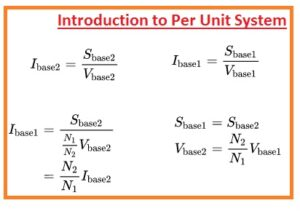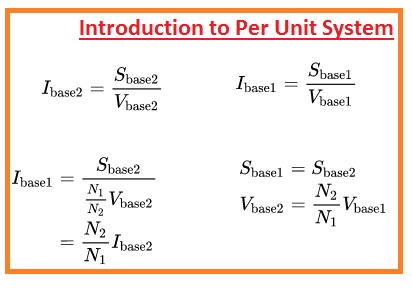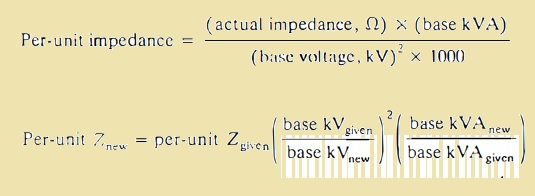The ratio between the real value of any element in the electrical system with the reference value of the same element having the same unit like the real or actual value has is called per unit system. It is unitless since both terms in the ratio are the same. This system is used to make calculation easy for different parameters like voltage power current.

It makes it easy for us to resolve the power system in a simple way since it consists of a large number or complicated calculations. In this post, we discuss different parameters used in this system and learn how this system helps us to make power system easy for us. So let get started

Introduction to Per Unit System

• Normally voltage transmitted in an electrical power line or transmission line is taken in kilovolts. For power transmission units used are MW, KVA MVA.
• these terms are used in form of a per-unit system
• Suppose that we have a transmission lien through which base value of voltage passing is one twenty volts then the voltage value of one zero eight, one twenty, and one twenty kilovolts will be transformed in 0.9, 1, and 1.05 in per-unit system conversion.
• The ratio between any electrical quality to its base value is called per unit quantity and it defines in form of point or percentage.
• the use of a per unit system is easy to understand then the actual value of system parameters like volts, current, and ohms.
• Ther is such correlation among the current volts and power that and impedance that if we choose base parameters for any two will give the results about other two-parameter
• the base impedance is such value that has voltage loss same to the base volts in case current is passing impedance has base value of current
• the value of base KVA in single-phase system is multiple of base KV and base ampers.
• generally, base MVA ann base volts in KV are taken as base.
• In case of a single or three-phase system in which current is referred as line current voltage as neutral and KVA as KVA per phase then these expressions are used

Base current in ampere=base kVA/base voltage, kVLN

Base impedance in ohms=base voltage VLN/base current

base impedance in ohms=base current

(Base current KVLN)2x 1000/base KVA

Base impedence=(Base current KVLN)2/MVA

Base power KW=Base KVA

Per unit system=actual impedance in ohm/base impedance in ohm• All these equations are design for single-phase system and term LN is line to neutral volts.
• KVLN are the volts about single phase in signal phase system or line to ground in case one end is ground.
• the balance three-phase system is discussed like single line having neutral as returning path the base parameters in the impedance circuitry are KVA per phase and KV for the line to neutral.
• Information is given as net three-phase KVA or MVA  and line to line KV
• Duet to the generalization of line to line volts and net KVA  problems comes to discuss the expression among the per unit of line volts and per unit of phase volts.
• Though the line volts are taken as base volts in a single phase.
• the base volts to neutral is base volts through-line to line volts divided with √3
• As it is ration among the lline to the line and line to neutral volts for balance three system the per unit term of line to neutral volts at the line to neutral volts base is equal to the per-unit term of line to line volts for simlt values at the line to line volts since our system is balance
• The equation for three-phase balance systems are mentioned here.
• Base kV A  = 60,000 kV A
• Base k VLL=120 kV
• Base KVA for singel phse =60,000/3=20000KVA
• base KVLN=120/√3=69kv
• in cae of real line to line volts of 108KV in balance THREE PHASE  system line to neutral volts will be
• 108/√3=62.3x
• so per unit volts will be
• 108/120=62.3/69.2=0.90
• So in the three-phase system for per unit values, final expression will be
• Base current tin ampere=base kYA /√3xbase volts KVLL
• base impedence=base volts, KVLL.√3)2x1000/base KVA/3

### How to Change the Base of Per Unit System

• give below formula are used to change base of per-unit system